# Python - 循环结构

## 🐍While循环

While循环的语法格式如下

while 条件表达式 ：
代码段（包含改变计数器值的语句）


while 关键词后面紧跟的条件表达式决定了循环是够进行下去。

count = 1		#计数器
sum0 = 0		#统计和值，初始值为0
while count <= 100 :
sum0 += count
count += 1
print(sum0)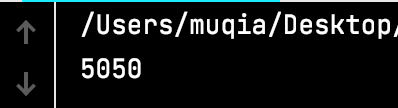While循环的语法要点需要做的就是以下三件事：
1、定义计数器的初始值，这个计数器可以计数，也可以用作求解问题的数字处理。
2、While循环条件保证计数器在一定条件下退出循环。
3、循环做的事情放在循环体里，同是不要忘记计数器的叠加效果。

## 🐍While…else…循环

While…else…的语法格式如下：

while 条件表达式 ：
代码块1
改变计数器的值
else ：
代码块2


count = 1
while count < 3 :
print("密码正确，您可以取款了")
break
else :
input("密码错误，请再一次输入密码：")
count += 1
else: print("密码错误，卡被吞")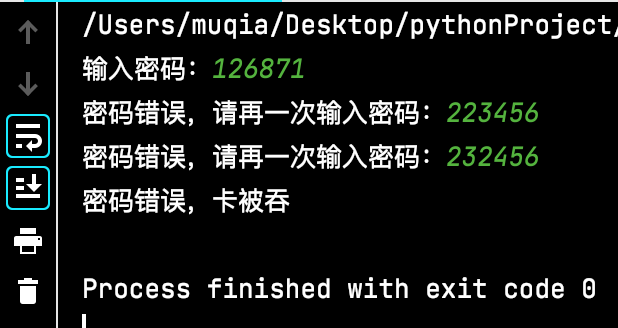break用来推出循环体，不再执行本层循环。

## 🐍for循环

while循环和for循环用于处理不同的问题，while应用在不知道循环次数的情况下，for应用在确定循环次数的情况下。
for循环语句的格式如下：

for 临时变量 in 可迭代对象 ：
循环体


sum0 = 0
for count in range(1,101,1) :
sum0 += count
print(sum0)


range函数可以接受3个参数，range（start，stop，step）产生一个从start开始到stop结束的一个整数序列，step是步长。

## 🐍for…else…循环

While…else…的语法格式如下：

for 临时变量 in 可迭代对象 ：
循环体
else ：
代码块2


password = input("输入密码：")
for i in range(1,3,1) :
print("密码正确，您可以取款了")
break
else :
input("密码错误，请再一次输入密码：")
else: print("密码错误，卡被吞")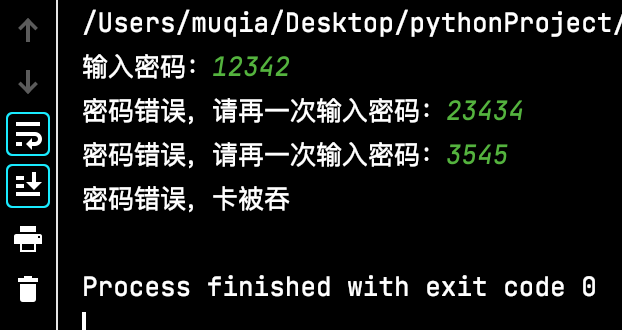## 🐍循环体结束语句

Python的循环逻辑控制是依靠for和while这两种语句来实现的。但是，有时不需要把循环执行完，条件合适时需要直接退出循环，这时可以使用break语句。还有一种需求，不需要退出整个循环，而是退出本次循环，可以使用continue语句。这两个语句的区别如下：
1、break用于完全结束一个循环，跳出循环体之行循环后面的语句。
2、continue只是终止本次循环，接着还之行后面的循环。

• 循环打印奇数

for i in range(1,11) :
if i%2==0 :
continue
print(i)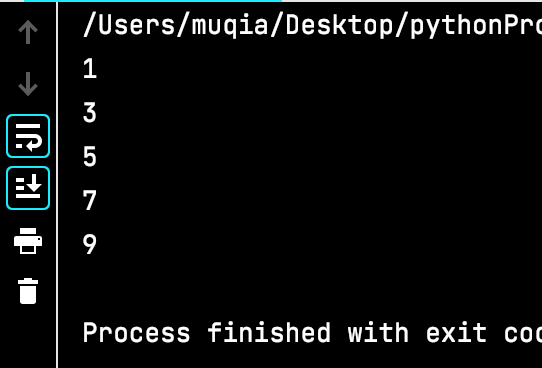• 循环打印闰年

for year in range(2020,2051) :
if year%4==0 and year%100!=0 or year%400==0 :
print(year)
break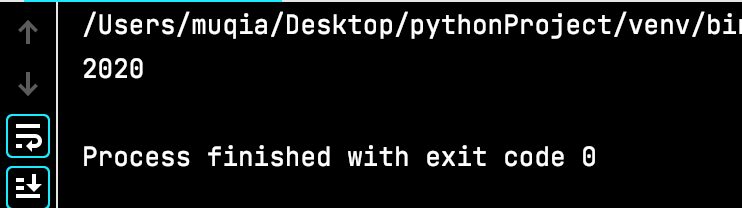## 🐍嵌套循环

1 * 1 = 1
1 * 2 = 2	2 * 2 = 4
1 * 3 = 3	2 * 3 = 6	3 * 3 = 9
1 * 4 = 4	2 * 4 = 8	3 * 4 = 12	4 * 4 = 16
1 * 5 = 5	2 * 5 = 10	3 * 5 = 15	4 * 5 = 20	5 * 5 = 25
1 * 6 = 6	2 * 6 = 12	3 * 6 = 18	4 * 6 = 24	5 * 6 = 30	6 * 6 = 36
1 * 7 = 7	2 * 7 = 14	3 * 7 = 21	4 * 7 = 28	5 * 7 = 35	6 * 7 = 42	7 * 7 = 49
1 * 8 = 8	2 * 8 = 16	3 * 8 = 24	4 * 8 = 32	5 * 8 = 40	6 * 8 = 48	7 * 8 = 56	8 * 8 = 64
1 * 9 = 9	2 * 9 = 18	3 * 9 = 27	4 * 9 = 36	5 * 9 = 45	6 * 9 = 54	7 * 9 = 63	8 * 9 = 72	9 * 9 = 81


for i in range(1,10) :
for j in range(1,10) :
print("%d*%d=%d"%(i,j,i*j))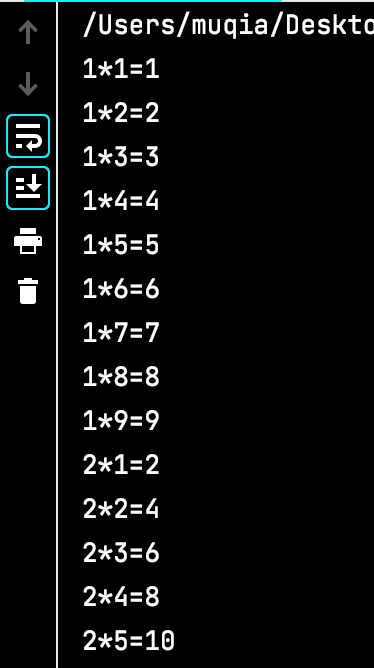for i in range(1,10) :
for j in range(1,10) :
print("%d*%d=%d"%(i,j,i*j),end="\t")
print()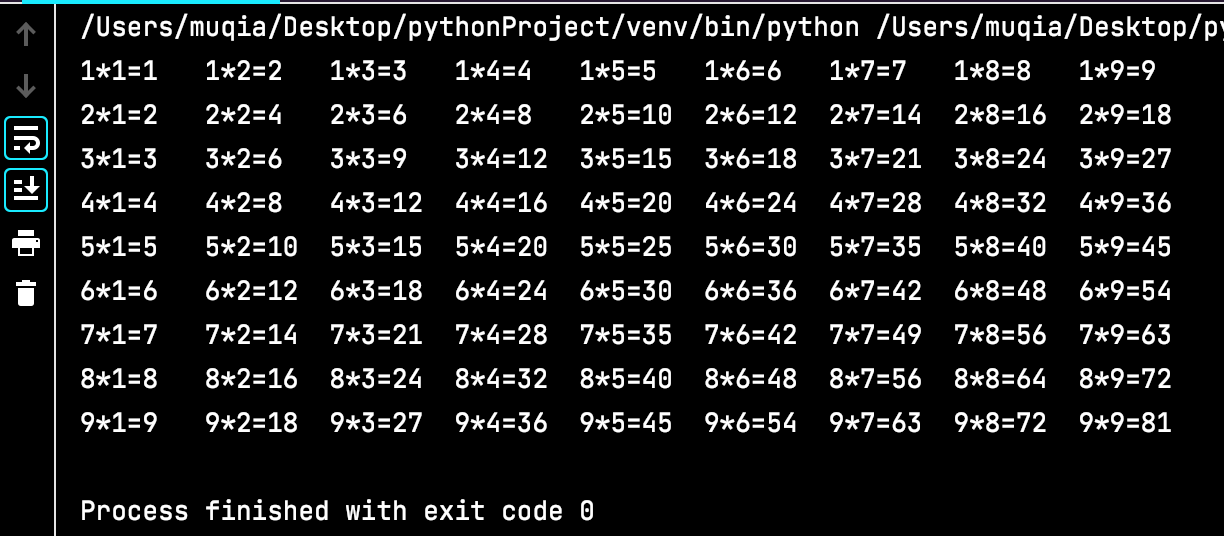for j in range(1,10) :
for i in range(1,10) :
if i > j :
continue
print("%d * %d = %d"%(i,j,i*j),end="\t")
print()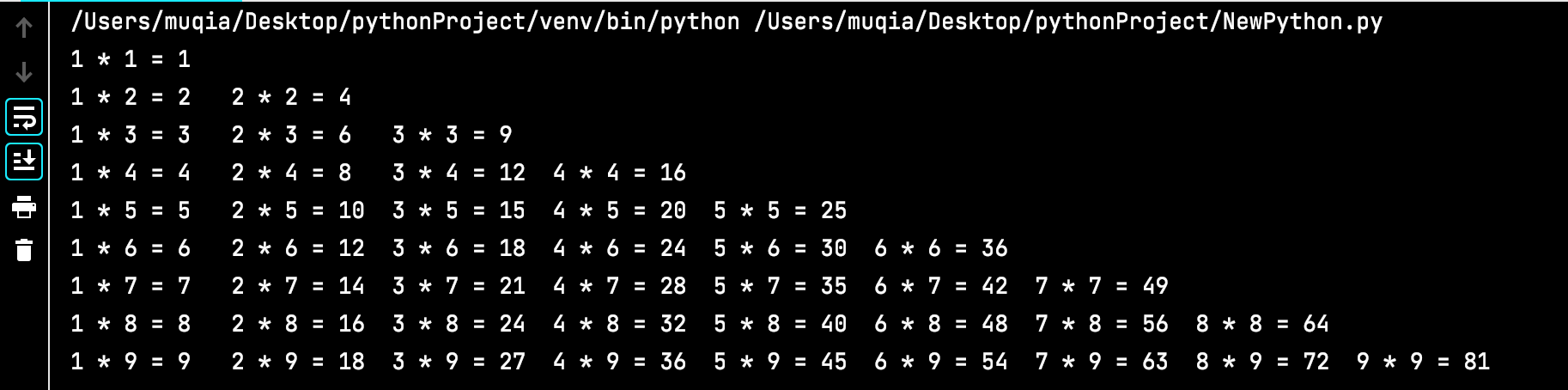04-13
09-172万+
04-173789
05-148742
03-061万+
04-293890
11-24866
03-203696
11-203155
02-222万+
08-061850
11-20928
03-281533
11-221万+

### “相关推荐”对你有帮助么？

•非常没帮助
•没帮助
•一般
•有帮助
•非常有帮助被折叠的  条评论 为什么被折叠?到【灌水乐园】发言¥1 ¥2 ¥4 ¥6 ¥10 ¥20获取中扫码支付点击重新获取扫码支付1.余额是钱包充值的虚拟货币，按照1:1的比例进行支付金额的抵扣。
2.余额无法直接购买下载，可以购买VIP、付费专栏及课程。余额充值